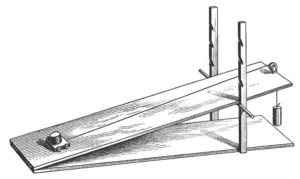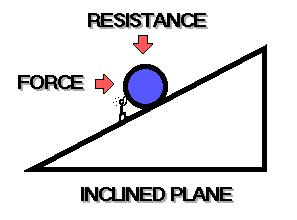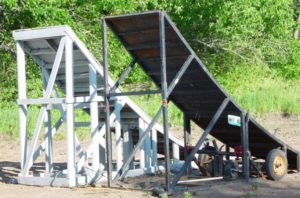Lesson 4A: An Engineering Introduction to Machines–ALTERNATE, Inclined Plane (December 14)

This SUPPLEMENTAL assignment is for ONLY for the students who have more than one engineering class with this teacher.

Objectives: (1) To introduce students to the basic concepts of machines, as used by engineers;  (2) to specifically learn the most basic principles of the inclined plane; (3) to practice concisely articulating a complex concept.

[/color-box]INCLINED PLANES

Based on Mr. Tunon’s notes and powerpoint1• Inclined plane, a simple machine consisting of a sloping surface, whose purpose is to reduce the force that must be applied to raise a load.
• To raise a body vertically a force must be applied that is equal to the weight of the body.
• The amount of work done (i.e., energy expended) in raising the body is equal to its weight times the distance through which it is raised.
• By means of an inclined plane a force smaller than the weight of the body can be exerted over a distance greater than the direct vertical distance, doing work equal to the product of the force and the distance through which it acts.
• If friction is ignored, the work done using the inclined plane will be exactly equal to the work done in lifting the body directly.
QUESTION 1 out of 20 (Include the question in your answer):   Define “inclined plane.”

QUESTION 2 out of 20 (Write the question and answer):   How much force must be applied to raise an object vertically.

QUESTION 3 out of 20 (Write the question and answer):   The work done (ignoring friction) using an inclined plain is equal to the weight of the object times  ______________.

• In any real system some work is done to overcome friction between the plane and the load. The actual mechanical advantage of an inclined plane is the ratio of the load lifted to the force applied; ideally it is equal to the ratio of the length of the sloping plane to its vertical rise.
• An inclined plane whose sloping length is 5 m and whose vertical rise is 1 m has a mechanical advantage of 5; a 300-newton load can be moved up such a plane by a 60-newton force.
QUESTION 4 out of 20 (Write the question and answer):   An inclined plane whose sloping length is 100 feet and whose vertical rise is 10 feet has a mechanical advantage of _______.
• The inclined plane has been modified in many ways. The screw and wedge are applications of the principle of the inclined plane but do not require that the load be moved vertically for their successful operation. The chisel, carpenter’s plane, auger bit, and ax are some of the many tools based on this principle.
QUESTION 5 out of 20 (Incorporate the question in your answer):   Give 5 examples of inclined plane or wedge, OTHER THAN than the examples in the preceding bullet-point.• The Mechanical advantage(ratio of load to effort) of the inclined plane equals to length of the plane over the height of the plane, in a case where efficiency is 100%.
• To calculate the mechanical advantage of an inclined plane, divide the length of the ramp by the height of the ramp. M.A=Length of the sloping surface / Height of the plane.QUESTION 6 out of 20 (Incorporate the question in your answer):   What information do you need in order to calculate the mechanical advantage of a ramp?

QUESTION 7 out of 20 (Write the question and answer.  Please show your calculations):   Assume the length of ramp is 150 feet.  The height of the ramp is 40 feet.  What is the Mechanical Advantage?

Ramps/ Inclined Planes

• Ramps are sometimes known as inclined planes or wedges.
• The head of an axe is a wedge working in a different way. An axe forces wood apart in two ways. The handle works like a lever, magnifying the force you apply. The wedge-shaped blade concentrates the force over a smaller area, increasing the pressure on the wood and splitting it apart. The blade of a knife works the same way.
QUESTION 8 out of 20 (Write the question and answer):   Ramps, inclined planes and wedges all refer to the same basic type of simple machine (TRUE or FALSE?)

QUESTION 9 out of 20  (Incorporate the question in your answer):   Explain how an axe works–You may paraphrase the description in the bullet point above, but don’t just copy the language.

QUESTION 10 out of 20  (Incorporate the question in your answer):  Explain  why ramps were essential to some ancient building projects.

The purpose of the next question is to encourage you to express an idea clearly and concisely.

QUESTION 11 through 20.  In EXACTLY 10 words (NOT 9 and NOT 11) convey the most important thing to know about the inclined plane.

1.   John Tunon J. P. Taravella High School